# Symmetry of Quasicrystals

by Ron Lifshitz

We saw in the introduction that the facets of a (quasi)crystal as well as its diffraction diagram clearly reveal a certain kind of symmetry. This symmetry is expressed by the set of rotations that leave the directions of the facets unchanged (Figure 1), or the set of rotations that leave the positions of the Bragg peaks in the diffraction diagram unchanged (Figure 2). But what exactly is the nature of the symmetry exhibited by the microscopic arrangement of atoms in the crystal itself? What do we really mean when we say that a crystal has the symmetry of a certain rotation?

Figure 1. Scanning electron micrographs of single grains of quasicrystals: (a) an Aluminum - Copper - Iron alloy which crystallizes in the shape of a dodecahedron. This is one of the five Platonic solids, containing 12 faces of regular pentagons. Its symmetry is the same as that of the icosahedron, one of the other platonic solid with 20 faces of equilateral triangles. (b) An Aluminum - Nickel - Cobalt alloy which crystallizes in the shape of  a decagonal (10-sided) prism. Photographs courtesy of An Pang Tsai, NRIM, Tsukuba, Japan.Figure 2. Typical diffraction diagram of a quasicrystal, exhibiting 5-fold or 10-fold rotational symmetry. Source unknown.

In the case of a periodic crystal we mean that the rotation leaves the density of the crystal invariant to within a translation. This means that after rotating we may need to apply a translation but after that the points of the rotated crystal will exactly coincide with the points of the original crystal. Consider for example a 4-fold rotation of Escher's angel and devil drawing (Figure 3). The densities of quasiperiodic crystals, however, in general possess no such symmetries. In fact, it is a nice exercise to show that if a two-dimensional crystal contains more than a single point, about which an n-fold rotation (n>2) brings it into perfect coincidence with itself, then the crystal is necessarily periodic. So a crystal with, say, 5-fold symmetry cannot contain more than a single point of "exact" 5-fold symmetry, and if we were given a finite chunk of that crystal there is a good chance that we would never find that point. We can therefore no longer rely on the criterion of "invariance to within a translation" as a definition of crystal symmetry. Nevertheless, certain rotations, when applied to a quasiperiodic crystal, take it into one which looks very much like the original unrotated crystal.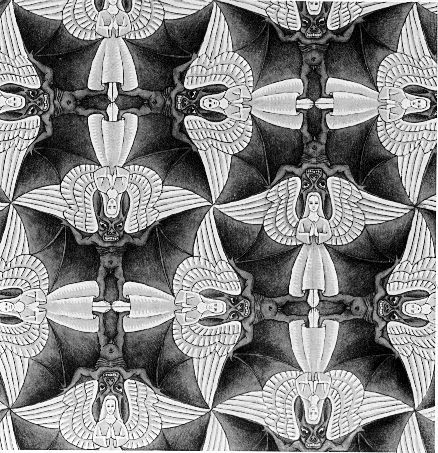Figure 3. A patch of M. C. Escher's drawing, containing infinitely many points of exact 4-fold symmetry. © Copyright Cordon Art B.V.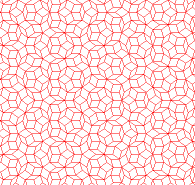Figure 4. A patch of the Penrose tiling, rotating about its single point of exact 5-fold symmetry. © Copyright Roger Penrose.
To demonstrate this we shall use the Penrose tiling (Figure 4) as our model of a two-dimensional quasicrystal. The tiling contains two kinds of rhombic (diamond shaped) tiles  a thin one with a small angle of 36 degrees and a thick one with a small angle of 72 degrees. These two types of tiles are arranged in a very specific and ordered manner to produce a tiling which is quasiperiodic. If we were to place atoms at, say, the vertices of the tiling and perform a diffraction experiment, we would see a diffraction pattern similar to the one in Figure 2 above. In the next section you can read a little more about generating these kinds of tilings and even create your own tilings using an interactive Java program.

This is a good point to clear up a widespread misconception about the Penrose tiling. If you wait long enough for your browser to download the entire image file you will see the Penrose tiling in Figure 4 beginning to rotate. [Clicking your browser's "stop" button will freeze the animation. Clicking the "reload" button will get it going again.] The point of rotation is the one and only point in the whole infinite tiling which has "exact" 5-fold symmetry. Nevertheless, due to the existence of this single point many people tend to think that the Penrose tiling has only 5-fold symmetry, whereas in fact it has 10-fold symmetry. It is a decagonal and not a pentagonal tiling, as we shall immediately explain, even though it contains not even a single point of "exact" 10-fold symmetry.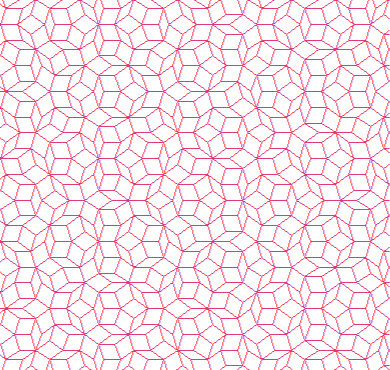Figure 5. The 10-fold symmetry of  the Penrose tiling: Coincidence of bounded regions.
To see the effect of a 10-fold rotation on the Penrose tiling take a look at the animation in Figure 5. A red Penrose tiling is placed over an identical blue one. The red tiling is then rotated counterclockwise. Nothing interesting happens until a 10-fold (36 degree) rotation is completed and a fair fraction of the vertices of the two tilings coincide. The red tiling is then translated by some amount until whole regions, of about 10 to 15 tiles across, coincide. Finally, the red tiling is translated even further until regions of the order of the full patch coincide. Between the coinciding regions there always remain strips, often called "worms," containing tiles that do not match.  If we could see the entire infinite tiling we would observe that any bounded region in the rotated tiling can be found in the unrotated tiling, but the larger the region the further away we have to look in order to find it. Even so, there is no translation that will bring the whole infinite red tiling into full coincidence with the infinite blue one.

The reason for this observation is that the two tilings contain the same statistical distribution of bounded substructures of arbitrary size. This property is demonstrated by Figure 6 below which shows patches of two Penrose tilings that differ by a 10-fold rotation. Both patches contain roughly the same number of 5-fold stars pointing to the right (highlighted in yellow) and roughly the same number of such stars that are surrounded by five thin tiles (highlighted in magenta). To observe the statistics of larger structures we would need to look at larger patches, but if we could do that we would find that the two tilings contain the same statistical distribution of bounded structures at all scales.

Figure 6. The 10-fold symmetry of  the Penrose tiling:  Statistics of bounded structures.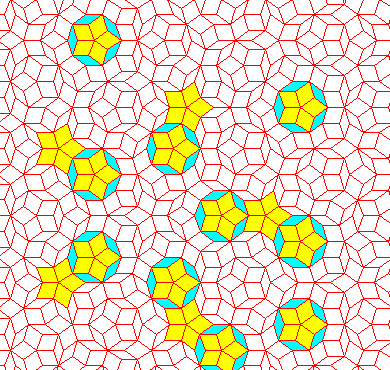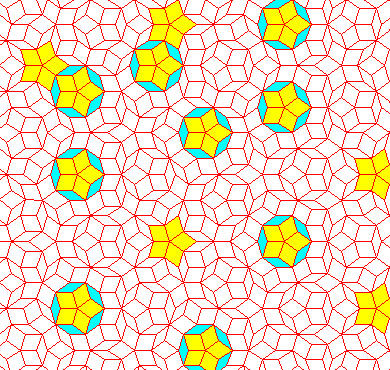Two crystals whose densities are statistically the same in this sense are called indistinguishable, a term which was coined by Dan Rokhsar, David Wright, and David Mermin. The key to understanding the symmetry of quasicrystals is to realize that a symmetry operation is one that takes the crystal into an indistinguishable one, just like the 10-fold rotation does to the Penrose tiling. Only when the crystal is periodic does the notion of indistinguishability reduce back to the traditional notion of "invariance to within a translation."

## Point groups and space groups

The collection of all rotations and reflections that are symmetries of a given crystal form the point group of the crystal. The word "group" refers to a particular mathematical structure, which in this context simply states that (1) If a clockwise n-fold rotation is a symmetry then so is a counterclockwise n-fold rotation; and that (2) The effective operation you get by applying
two symmetry operations one after the other is also a symmetry operation.

To fully specify the symmetry of the crystal  given by what is called the space group of the crystal  we need to indicate exactly in what way the original and rotated versions of the crystal differ. If the crystal is periodic, where indistinguishability reduces to invariance to within a translation, all we need is to specify exactly what translation needs to follow each rotation to leave the crystal invariant. If the crystal is quasiperiodic a simple translations are insufficient and the required information is given by a set of very simple functions, called "phase functions." Alternatively, one may also express the information contained in a phase function by a "super"-translation  a translation in an abstract high-dimensional space called "superspace." We prefer the first, more direct, approach but there are also advantages to the high-dimensional description. For more detail please refer to the suggested reading below.

Please visit the next section on quasiperiodic tilings, where you can create your own tiling using an interactive Java program, and the section after that which deals with color symmetry and magnetic symmetry of quasicrystals.

#Suggested Further Reading

1. A good informal introduction to crystallography:

2. M. Senechal, "Crystalline symmetries: An informal mathematical introduction" (Adam Hilger, Bristol, 1990).
3. An introductory article:

4. R. Lifshitz, "The symmetry of quasiperiodic crystals," Physica A 232 (1996) 633-647.
5. A detailed review article:

6. N. D. Mermin, "The space groups of icosahedral quasicrystals and cubic, orthorhombic, monoclinic, and triclinic crystals," Reviews of Modern Physics 64 (1992) 3-49.
7. A chapter on the "superspace" description may be found in the textbook:

8. C. Janot, "Quasicrystals: A primer," 2nd edition (Clarendon Press, Oxford, 1994), ch. 3.

#Other Sites on Crystal Symmetry

• Commission on Aperiodic Crystals of the International Union of Crystallography.
• Special Interest Group on Aperiodic Crystals of the European Crystallographic Association.
• The Geometry Junkyard - Symmetry and group theory. [Mathematics and geometry.]
• Michael Baake, Tübingen University, Germany. [Symmetry and mathematics of aperiodic structures.]

• Note that Baake uses the term "Local Isomorphism" instead of "Indistinguishability."
• Charles Radin, University of Texas, Austin. [Symmetry of aperiodic tilings.]

• Note that Radin uses the term "Statistical Symmetry" instead of "Indistinguishability."
• Steffen Weber, NIRIM, Tsukuba, Japan. [Introduction, mainly structure and geometry.]
• Akiji Yamamoto, NIRIM, Tsukuba, Japan. [Crystallography, "superspace groups."]This page has been visitedtimes since February 20, 1999.